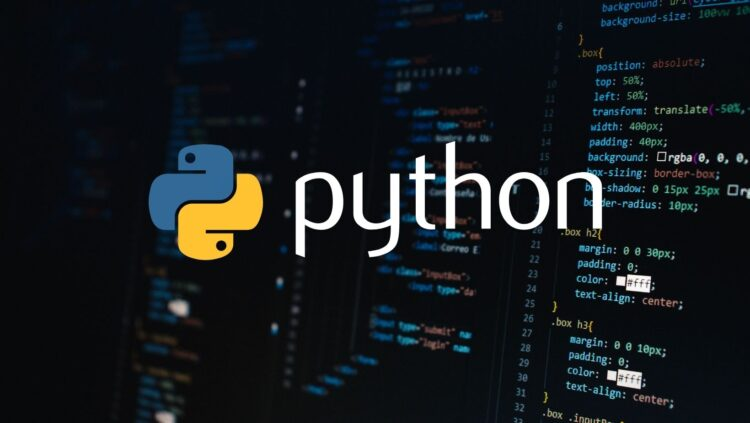# Python Program to Check Palindrome or not

#### Python Program to Check Palindrome or not

Write a Python Program to Palindrome checkPalindrome in Mathematics:

A palindrome is a sequence of characters or numbers that reads the same forwards as it does backwards. In the case of numbers, a palindrome number remains the same when its digits are reversed.

For example, 121, 1331, and 1221 are palindromic numbers.

Python Program to Check Palindrome:

Here’s a Python program that checks whether a given number is a palindrome or not:

``` num = int(input("Enter a number: ")) original_num = num reverse_num = 0 while num > 0:     digit = num % 10     reverse_num = reverse_num * 10 + digit     num //= 10 is_palindrome = original_num == reverse_num print("Palindrome" if is_palindrome else "Not Palindrome") ```

Explanation:

• The program starts by taking an integer input from the user using the input() function and converting it into an integer using the int() function. This input is stored in the variable num.
• The variables original_num and reverse_num are initialized with the original number entered by the user and 0, respectively.
• The program uses a while loop to reverse the digits of the input number:
• Inside the loop, the last digit of the number is extracted using the modulo operator % (num % 10), and it’s added to the reverse_num after shifting it one place to the left (multiplied by 10).
• The last digit is removed from num using integer division (num //= 10).
• After the loop, the program compares original_num with reverse_num to determine if the number is a palindrome. If they’re equal, the number is a palindrome; otherwise, it’s not.
• The program prints “Palindrome” if is_palindrome is True, indicating that the number is a palindrome. Otherwise, it prints “Not Palindrome“.

Output 1 (Palindrome):

```Enter a number: 121 Palindrome```

In the first example, the user enters 121, which is a palindromic number. The program reverses the digits and finds that the number is the same forwards and backwards, so it displays “Palindrome” as the output.

Output 2 (Not Palindrome):

```Enter a number: 123 Not Palindrome```

In the second example, the user enters 123, which is not a palindrome. The program finds that the reversed number is different from the original number, so it displays “Not Palindrome” as the output.